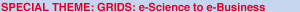ERCIM News No.45 - April 2001 [contents]

### Factoring Large Numbers on a Grid of Computers

by Herman te Riele and Walter Lioen

Probably the first grid computing project on Internet was initiated by Arjen Lenstra in 1988. The purpose was to answer the question: how large integers can we factor with our present algorithms? This question was, and is, of interest to cryptographers because any serious attempt to answer it gives crucial information about the security of the RSA public-key cryptosystem. This cryptosystem is used on a world-wide scale in secure communication. CWI has contributed actively to the state-of-the-art in factoring: as provider of algorithmic improvements, efficient implementations and a large computing grid, and as coordinator of the grid of people, computers and data storage resources which has led to the present world record: the factorization of a difficult 512-bit RSA modulus.

Factoring large numbers is a classical mathematical problem. The best methods available are complicated and extremely time-consuming, but the larger part of the computations can be split up trivially into smaller parts and distributed among as many processors as we like. Data transport is negligible compared with computing time. The most time-consuming part is a sieving step: essentially, on a large two-dimensional grid G of points (a,b), where both a and b run through a given set of consecutive integers, values of a polynomial P(x,y) have to be found consisting only of small prime factors. Such ‘smooth’ values are extremely scarce, but there is one property of polynomials which can facilitate this search considerably: if we know that some integer q divides some polynomial value P(a,b), we may add/subtract any multiple of q to/from a and b, and still obtain a polynomial value which has q as divisor. For example, for the quadratic polynomial P(x,y) = x2 - y2 +2xy - 3y + 1, we have P(2,2) = 3, so that, eg, P(2,5), P(5,2), P(5,5), P(-1,2), P(-1,5) all have a divisor 3. Now, the sieving consists of marking all grid points (2+3k, 2+3l) in G as having the prime divisor 3 without doing any trial division by 3. The larger G, the more efficient this sieving. This is carried out for all primes in a given set of small primes. Figure 3 illustrates this for the three primes 2, 3, and 5. Grid points marked by many small primes are selected as candidates for giving a smooth polynomial value. For the largest numbers which have been factored, the grid G contains between 1014 and 1015 grid points. This grid size is much too large to be handled by one processor of a modern computer, so it is split up into subgrids, which are distributed among different processors. Each processor receives the characteristics of a subgrid, sieves it in search of smooth polynomial values, and stores the values found. Processors are allowed to drop out, because what counts is the number of smooth values found, not which ones. The size of the grid G is chosen such that a drop-out of some computers in the network is taken into account.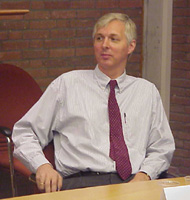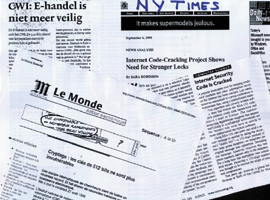Figure 1: Arjen Lenstra (Citibank, New York), one of the pioneers of the Grid on Internet. Figure 2: Breaking RSA-512 made international headlines.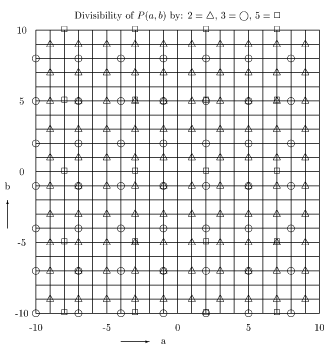Figure 3: 2D grid for the polynomial P(x,y) = x2 - y2 + 2xy - 3y + 1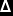means divisible by 2, grid points (1+2k, 1+2l)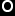means divisible by 3, grid points (2+3k, 2+3l)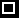means divisible by 5, grid points (2+5k, 0+5l)

Since 1994, CWI is running a ‘factory’: during night time and weekends the idle time of servers, workstations, and PCs is being used for carrying out the sieving step, needed to factor large integers. In our first approach, the factory was running continuously and we monitored the keyboard and mouse activity to stop the factorization processes. However, the user had to wait a couple of seconds for his machine to stop the factorization process, swap it out, and swap in his own process. After user complaints we ran the factory only during non-office hours. However, we still used the UNIX stop signal, and a large amount of swap space. So we still had complaints from people running out of swap space. Finally, we restarted jobs from the point where they stopped by looking at the already available output (as a sort of checkpoint). Workstation owners are able to stop the jobs by running a setuid program. By running our factorization jobs on UNIX machines at priority nice -9, we were able to secure sufficient CPU for them, and our current setup is running stable for years now. This factory has contributed to several factoring world records which have been established in 1999 and 2000 by an international grid of about ten sieving sites called ‘The Cabal’, coordinated by CWI. A second ERCIM participant in this project is INRIA Lorraine and Loria (Paul Zimmermann). The most appealing record was the factorization of a 512-bit RSA modulus: a number of 155 decimal digits. Since moduli of this size are used as actual RSA keys in E-commerce and communication protocols, this record attracted much publicity. One future aim in this project is to collect so much grid computing power, that it becomes feasible to factor a 640-bit RSA key within one year.